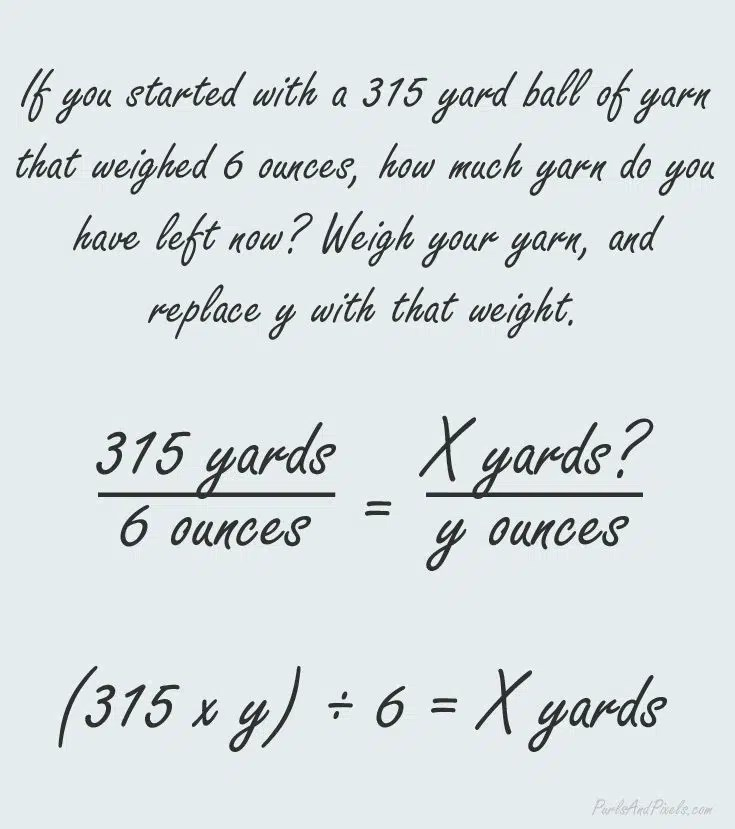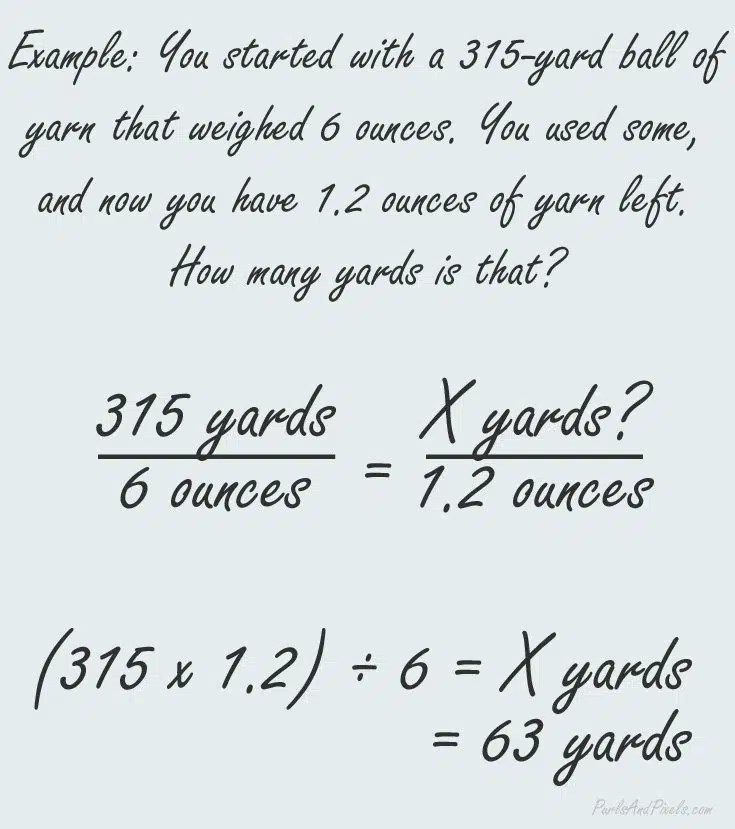# How to calculate yarn yardage using weight

###### A tutorial from Liz @PurlsAndPixels

Trying to find out how much yarn you need for a project can sometimes feel like a guessing game. Do you have enough? There is one way to be sure. Do a little yarn math.These are simple ratios that I remember learning in middle school, but whenever I come back to do them, I have to look it up again. I’m terrible with memorization. If you are too, bookmark this page and come back to it when you need a reminder. That’s what I’ll do!

## Measuring Yarn in Yards and Ounces

Most yarn is measured using ounces and yards, rather than feet and grams. We will use yards and ounces for the examples here, but these ratios would work just fine if you wanted to measure in another unit.

Nearly every ball of yarn you purchase new will have a label noting yardage and weight in ounces. After you use some of that yarn, you may want to know how much you have left. Lots of little projects are great for leftover yarn, but it helps to know a close estimate of yardage before you start. To find out, we will use a simple ratio to cross multiply, then divide to find out how much yarn is left.

To find out how much yarn you have left, you will first need to know the weight and length of yarn you started with. This will be written on the label of a new skein. You will also need a scale that weighs in ounces, so that you can get the current weight of your yarn.

Our basic math will use this formula:Here is an example: If you started with a ball of Caron Simply Soft Yarn, a common yarn found in a lot of places, you would have started with 315 yards and 6 ounces of yarn, as shown on the label.  After you’ve made a project or used some of your yarn, you weighed the leftovers to find that you have exactly 1.2 ounces of yarn left. How many yards is equal to 1.2 ounces of this yarn?

Start by filling in your formula with the length and weight of a full ball of yarn:Then solve the math problem by filling in the weight of the yarn you have left. In our example, replace y in the formula with 1.2 (the weight of the yarn you have left). To complete the math to find out your yarn yardage you will solve the ratio by cross multiplying and dividing. As shown below, you multiply the length of a full skein by the weight you have left (the number on the top left times the number on the bottom right). Then, divide the number you get by the weight of a full skein of yarn (the number on the bottom left).I hope you found this quick yarn math tutorial helpful. Pin it, bookmark it, or share it with your friends using the social links below. Happy crafting!